# Field

This article defines a property of integral domains, viz., a property that, given any integral domain, is either true or false for that.
View other properties of integral domains | View all properties of commutative unital rings
VIEW RELATED: Commutative unital ring property implications | Commutative unital ring property non-implications |Commutative unital ring metaproperty satisfactions | Commutative unital ring metaproperty dissatisfactions | Commutative unital ring property satisfactions | Commutative unital ring property dissatisfactions

## Definition

### Symbol-free definition

A field is a commutative unital ring with the additional property that its multiplicative group comprises all the nonzero elements, that is, with the property that all nonzero elements are invertible.

Alternatively, a field is a commutative unital ring with no proper nontrivial ideal.

### Definition with symbols

A field is a set$F$ endowed with constants$0$ and$1$ (not equal), a unary operation$-$ and binary operations$+$ and$*$ such that:

•$a + (b + c) = (a + b) + c$ for all$a, b, c$ in$F$
•$a + 0 = a$ for all$a$ in$F$
•$a + b = b + a$ for all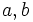$a, b$ in$F$
•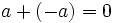$a + (-a) = 0$ for all$a$ in$F$
•$a * (b * c) = (a * b) * c$ for all$a, b, c$ in$F$
•$a * 1 = a$ for all$a$ in$F$
•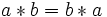$a * b = b * a$ for all$a, b$ in$F$
•$a * (b + c) = (a * b) + (a * c)$ for all$a, b, c$ in$F$
• For all nonzero$a$ in$F$, there exists a$b$ in$F$ such that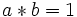$a * b = 1$

## Metaproperties

### Closure under taking subrings

This property of commutative unital rings is not closed under taking subrings; in other words, a subring of a commutative unital ring with this property need not have this property

A subring of a field need not be a field. It could be any integral domain. For instance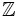$\mathbb{Z}$ is a subring of$\R$.

## Effect of property operators

### The subring-closure

Applying the subring-closure to this property gives: integral domain

## Module theory

Modules over fields are precisely the same as vector spaces. In particular any finitely generated module over a field is a free module and the number of generators is independent of the choice of generating set.